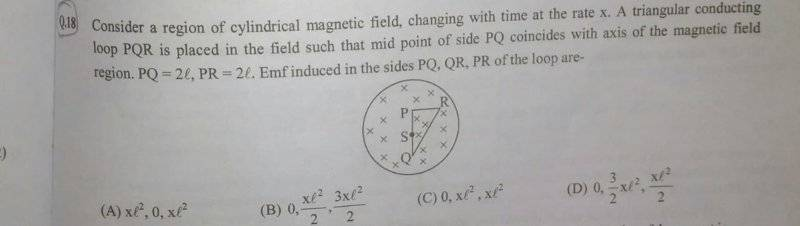# Challenging question about electromagnetic induction

## Homework StatementI have been stuck on this for weeks

∫E.dl = -dφ/dt

## The Attempt at a Solution

Total EMF (V) = -dφ/dt (Where φ is the magnetic flux through the loop)
⇒V = -A(dB/dt) (Since Area remains constant)
⇒V = -Ax (x=dB/dt)
⇒V = -2xl^2
I do not know how to proceed.

## Answers and Replies

haruspex
Science Advisor
Homework Helper
Gold Member
2020 Award
I'm no expert on this, and I can't find an online reference to support this idea, but maybe you can assume that as the field strength changes lines of flux move radially. That would show where no emf is produced. You can then cut the triangle in a certain way to figure out the remaining two emfs.

TSny
Homework Helper
Gold Member
I guess that the phrase "emf induced in the side PQ" means the magnitude of the integral ∫E.dl, where the integral is taken along the side PQ and E is the induced electric field.

By symmetry, what can you say about the shape of the induced electric field lines?
Can you use ##\oint##E.dl = -dφ/dt to determine the magnitude of E at any point within the magnetic field region?

Last edited:
kuruman
Science Advisor
Homework Helper
Gold Member
I agree with @TSny's assessment. I would also recommend that you write the electric field vector in Cartesian coordinates after you find it and before you do the line integrals.

rude man
Homework Helper
Gold Member
In the diagram, PQ >> PR yet it says they are the same length. Lousy drawing or typo?
Hint: don't evaluate the emf along QR explicitly! Use what post 3 says.

Last edited:
kuruman
Science Advisor
Homework Helper
Gold Member
In the diagram, PQ >> PR yet it says they are the same length. Lousy drawing or typo?
Not very good drawing. The triangle is supposed to be right isosceles.

•Naman Singh
rude man
Homework Helper
Gold Member
Not very good drawing. The triangle is supposed to be right isosceles.
That is my assumption also (but it's just an assumption). So we assume the given dimensions are gospel, not the drawing.

kuruman
Science Advisor
Homework Helper
Gold Member
That is my assumption also (but it's just an assumption). So we assume the given dimensions are gospel, not the drawing.
Yes. It appears that the drawing is not to scale. The problem clearly states that ##PQ=PR=2l##. The only assumption is that angle QPR = 90o. Without it there is no choice that matches the answer.

I guess that the phrase "emf induced in the side PQ" means the magnitude of the integral ∫E.dl, where the integral is taken along the side PQ and E is the induced electric field.

By symmetry, what can you say about the shape of the induced electric field lines?
Can you use ##\oint##E.dl = -dφ/dt to determine the magnitude of E at any point within the magnetic field region?

Thanks for the hints but I'm really not making headway with this. Can you please explain how symmetry plays a part here?

kuruman
Science Advisor
Homework Helper
Gold Member
Thanks for the hints but I'm really not making headway with this. Can you please explain how symmetry plays a part here?
Symmetry says that the electric field lines in the region of interest are concentric circles. What does this suggest about ##\int \vec E \cdot d\vec l## along segment PQ? What about along segment RS or any radial line segment?

Last edited: